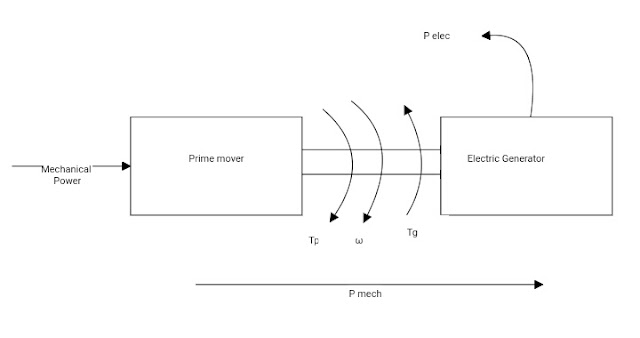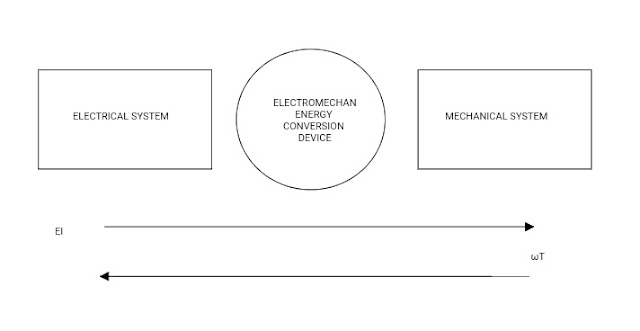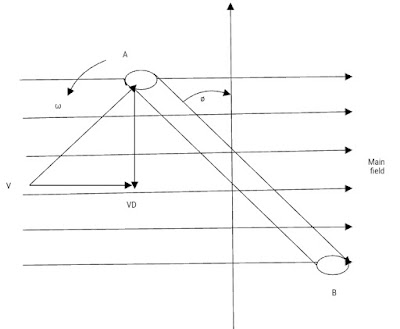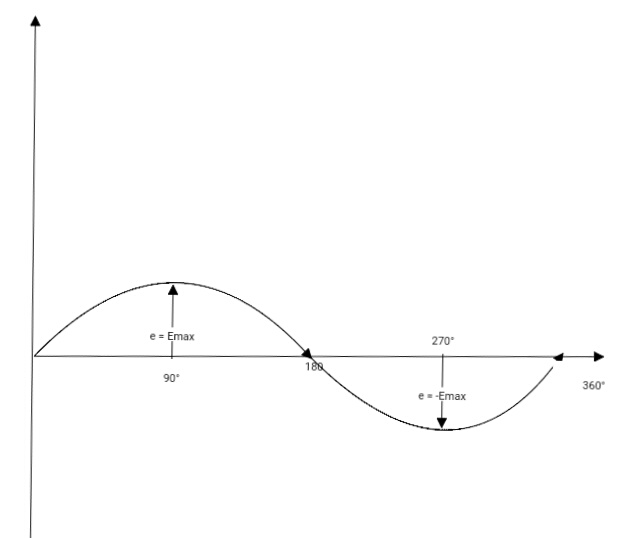### Ticker

6/recent/ticker-posts

# Principles of Electromechanical Energy Conversion

PRINCIPLES OF ELECTROMECHANICAL ENERGY CONVERSION

DYNAMO ELECTRIC MACHINE

A Dynamo is a machine that converts eighter Mechanical energy into Electrical energy or Electrical energy to Mechanical energy.

When a Dynamo is driven mechanically by a prime mover such as by a diesel engine, stream engine, stream turbine or water turbine and supplies Electrical energy, it is called a generator [ fig. 1 ].Fig. 1 Electric Generator

Prime mover Torque, Tpm = Generator Torque, Tg.

When the Dynamo draws Electrical energy from the supply mains and drives Mechanical devices such as line shaft and machine tools it is called a Motor [ fig.2 ].Fig. 2 Electric Motor
Motor Torque, T m = Load Torque, TL

In other words it can be started that the Generator converts Mechanical Power ωT into Electrical power EI and Motor converts Electrical power EI into Mechanical Power ωT.

This motoring and Generating action is basically reversible process i.e., the same machine is capable of Operating eighter as a generator or as a motor depending upon weather the supplied power is Mechanical or Electrical. This is illustrated in fig. 2.

However, in practice, devices may be Designed and constructed to suit one Particular mode of Conversion or the other.Block Reprensentation of Electromechanical energy conversion

An Electromechanical Energy Conversion device is a link between an electrical and Mechanical System and Electromechanical energy conversion needs the presence of natural phenomena which interrelate Electric and magnetic field on one hand and Mechanical force and motion on the other.

EMF Introduced in a coil Rotating in a Magnetic Field

Consider a single turn rectangular coil Rotating in counter - clockwise direction with angular velocity ω radians per second in a uniform Magnetic Field of flux density B Tesla's ( wb/m²), as illustrated.

When the coil is rotated in a uniform Magnetic Field with uniform angular velocity, an EMF is induced in its coil sides.

The magnitude of EMF Induced in the coil depends on

1) The flux density of the main magnetic field, B Teslas

2) The effective length of the coil side in the Magnetic Field, l meters

3) Number of turns on the coil, N

4) Velocity of the coil side perpendicular to the field, Vp meters/second and is given by the expressionFig. 3

e  =  2NBlVp Volts

(  ∵ Two coil sides are Connected in Series )

The coil sides do not always cut the line of flux in perpendicular direction while rotating in a Magnetic Field.

The component of velocity of the coil perpendicular to the field is Vp = V sin θ , Where v is the peripheral velocity of the coil and θ is the angle between the conductor and line perpendicular to the Magnetic axis fig. 3.

So the EMF Equation for the Generated be voltage becomes

e  = 2NBlv sin θ Volts

The magnitude of induced EMF can be determined if the values of N, B, l, v sin θ are known.

The value of the Induced EMF varies in magnitude and direction according to the instantaneous position of the coil relative to the Magnetic Field. The Cross sectional view of the coil and it's different positions at different instants are shown in fig.4

When the coil is in vertical position as shown in fig.4Fig.4 (a)Fig. 4 (b)Fig.4 (c)Fig. 4.(d)Fig. 4 (e)

a), (c) and (e) , no EMF is induced in the coil sides as the coil sides are moving parallel to the Magnetic Field and the rate of cutting flux is zero.

When the Coil is horizontal and moving at Right angle to the Magnetic Field, as shown in fig. 4 (b) and (d) the magnitude of EMF Induced in the Conductors A and B is maximum because the rate of cutting flux is maximum.Fig. 4

The direction of EMF Induced in Conductor A at instant shown in fig. 4. (b) is outward and at instant shown in fig.4 (d) is inward, as determined by Fleming's right hand Rule.

The direction of EMF Induced in Conductor B is opposite to that of Conductor A, as various from figs. The wave shape of the EMF induced in the coil is also shown fig. 4.

From the above discussion as well as from Eq. (2) it is obvious that the waveform of the Induced emf is Sinusoidal.